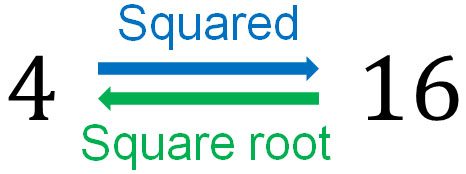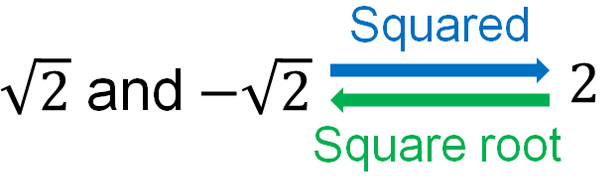An important area to learn in mathematics is square roots. In mathematics, we calculate powers; we multiply numbers by squaring or cubing them. Square roots are a relative of powers. If you have learned the concept of powers, you can understand square roots.

Square roots also use a symbol called root symbol (or radical symbol). In numbers that use roots, they are represented as $\sqrt{2}$ and so on. After studying square roots, you will see so many problems that use roots.

So what is the concept of square roots? And how can we use roots? What is the relationship between square roots and powers?

In addition, there are two types of numbers: rational numbers and irrational numbers. Numbers with a root are classified as irrational numbers. In most cases, we learn the concept of irrational numbers when we learn a root. Including these definitions, we will discuss the concept of a square root.

## What Is the Square Root: the Concept of Squaring a Number

First, what is the square root? In mathematics, we learn about powers. For example, 42 is $4×4=16$. Also, 43 is $4×4×4=64$. Power has the same meaning as multiplication.

In mathematics, on the other hand, there is the opposite of powers. Square root, which can be understood as the opposite of power. When squaring a number to get a specific number, it is called square root.

The answer to 42 is 16, as mentioned above. So what is the square root of 16? Since 16 is 42, the square root of 16 is 4. The square root is to be understood as the inverse of a square.Just note that in square root, there are two answers; in the calculation of squares, there is only one answer. In the square root, on the other hand, there is more than one answer.

What are some numbers that can be squared to get 16? As mentioned above, 4 squared is 16. However, squaring -4 also gives us 16. The answer to (-4)2 is 16. In other words, the square root of 16 is 4 and -4. Understand that in square root, there are two answers.

### How to Use the Square Root Sign

How can we express square roots? To represent a square root, we use the root symbol. For example, how can we represent the square root of 16? If we want to represent the square root of 16, we write $\sqrt{16}$ in mathematics; if squaring it and get 16, we can write $\sqrt{16}$.

However, 16 is 42. Therefore, we can remove the root symbol as follows.

• $\sqrt{16}=\sqrt{4^2}=4$

Similarly, the answer to $\sqrt{25}$ is 5. The answer to $\sqrt{100}$ is 10. By removing the root sign, we can express the number before squaring it.

• $\sqrt{25}=\sqrt{5^2}=5$
• $\sqrt{100}=\sqrt{10^2}=10$

-How Do We Think About Numbers That Cannot Be Squared?

However, there are many numbers that cannot be squared. For example, 36 is 62, so we can understand that the answer to $\sqrt{36}$ is 6. On the other hand, what is the square root of 2 or 3?

We mentioned earlier that we use root symbols to describe the numbers before squaring them. In the same way, for numbers 2 and 3, we can express the square root of 2 and 3 by using the root symbol. In other words, the square root of 2 is $\sqrt{2}$ and $-\sqrt{2}$.

When expressing square roots, be sure to use the root symbol. For example, the square root of 11 is $\sqrt{11}$ and $-\sqrt{11}$. And the square root of 15 is $\sqrt{15}$ and $-\sqrt{15}$. By using a root sign, we can represent the square root.

-Squaring a Number Will Always Remove Root Sign

The number before squaring is the square root. Therefore, when we square the square root, the radical symbol will always be removed. For example, we have the following.

• $(\sqrt{5})^2=5$
• $(\sqrt{11})^2=11$

### Representing the Radical Symbol as a Positive and Negative Number

Just as mentioned earlier, there are two answers to square root. However, in mathematics, positive numbers and negative numbers have opposite properties. Therefore, we must clearly distinguish whether they are positive or negative.

So we express the positive number of the square root as $\sqrt{a}$. On the other hand, the negative number is represented as $-\sqrt{a}$. For example, the square roots of 2 are $\sqrt{2}$ and $-\sqrt{2}$.

Why can the square root of 2 be expressed as $-\sqrt{2}$? It’s because when we multiply the negative by the negative, we get a positive result. We get the following.

• $(-\sqrt{2})^2=2$

In square roots, there is a rule to write the following.

• Positive square root: $\sqrt{a}$
• Negative square root: $-\sqrt{a}$The square root has two answers. When writing the answer in the square root, be sure to include both positive and negative numbers.

-Representing Numbers Without Square Roots

Note that if you can represent a number without a root sign, be sure to remove the radical sign. For example, what is the square root of 9?

When writing the square root of 9, $\sqrt{9}$ and $-\sqrt{9}$ are not the answer because 9 is 32. Therefore, we have to remove the radical symbol. The following is the correct answer.

• $\sqrt{9}=\sqrt{3^2}=3$
• $-\sqrt{9}=-\sqrt{3^2}=-3$

If it can be written without a root sign, we must remove the radical symbol.

### Approximate Value of $\sqrt{2}$ and $\sqrt{3}$

A number that becomes an integer by squaring is the square root. But the square root cannot be expressed as a specific number. In exceptional cases, such as $\sqrt{9}$ and $\sqrt{16}$, which contain squares, the number can be made into an integer by removing the root sign. However, in the case of $\sqrt{2}$ and $\sqrt{3}$, it is not possible to write an integer.

Instead, you can write an approximate value. A number that is not an exact number, but is very close, is called an approximate value.

For example, pi is 3.1415… and continues to infinity. However, in mathematics, we learned that pi is calculated at 3.14 as an approximation. In the same way, the square root can be written in approximations. For example, we have the following.

• $\sqrt{2}=1.4142…$
• $\sqrt{3}=1.7320…$
• $\sqrt{5}=2.2360…$

For example, 1.4 squared is 1.96. 1.5 squared is 2.25. We can see that the approximate value of $\sqrt{2}$ is between 1.4 and 1.5. Calculating these in detail, we get $\sqrt{2}=1.4142…$.

However, the approximations go on indefinitely. Therefore, it does not make sense to remember the approximate value of the square root. However, it is good to remember the numbers for the following.

• $\sqrt{2}≈1.41$
• $\sqrt{3}≈1.73$

In math, $\sqrt{2}$ and $\sqrt{3}$ are frequently used in calculations. It is also useful when reviewing the answers you have calculated to see if they are correct. Even when learning advanced mathematics, such as high school mathematics, the approximations of $\sqrt{2}$ and $\sqrt{3}$ are useful as knowledge.

There is no sense in memorizing approximations such as $\sqrt{5}$ and $\sqrt{6}$. On the other hand, $\sqrt{2}$ and $\sqrt{3}$ are useful if you remember the approximations.

## Rational and Irrational Numbers: Integers, Finite Decimals, Recurring Decimals Are Rational Numbers

When learning square roots, there is a word we learn at the same time: irrational numbers. There are several categories in mathematics, one of which is rational numbers and irrational numbers.

Rational numbers are numbers that can be expressed by fractions. Rational numbers include integers and decimals. An integer is one of the fractions, as shown below.

• $3=\displaystyle\frac{3}{1}$
• $5=\displaystyle\frac{5}{1}$

Decimals can also be expressed in fractions. It is as follows.

• $0.2=\displaystyle\frac{2}{10}$
• $1.23=\displaystyle\frac{123}{100}$

Decimals that do not continue infinitely are called finite decimals. 0.2 and 1.23 are finite decimals. The numbers 0.2 and 1.23 have an end.

Fractions, on the other hand, can have numbers that go on indefinitely. For example, the following.

• $\displaystyle\frac{10}{3}=3.33333…$
• $\displaystyle\frac{13}{7}=1.85714…$

In these fractions, the numbers go on indefinitely, but the same number is repeated. For example, $\displaystyle\frac{13}{7}$ is 1.85714285714285… and the same number will be repeated. Such regular decimals that go on indefinitely are called recurring decimals (or repeating decimals).

Decimals with regularity can be expressed as fractions. Therefore, a recurring decimal is a rational number. In short, the following are rational numbers.

• Integers
• Finite Decimals
• Recurring Decimals

In any case, understand that the numbers that can be expressed in fractions are rational numbers.

### Pi and Square Root Are Irrational Numbers

On the other hand, numbers that continue indefinitely but have no regularity cannot be expressed as fractions. Such numbers are called irrational numbers. Irrational numbers include pi $(\pi)$ and square root. These numbers are not regular, as shown below.

• $\sqrt{2}=1.4142135…$
• $\sqrt{3}=1.7320508…$
• $\pi=3.14159265…$

A number that is not a rational number is called an irrational number. Rational numbers can be expressed as a fraction, while other numbers are irrational. In short, the numbers that are not regular and cannot be represented by a fraction are irrational numbers.

Note that not all square roots are irrational. For example, $\sqrt{4}$ is a rational number. The reason is that $\sqrt{4}$ is 2, as shown below.

• $\sqrt{4}=\sqrt{2^2}=2$

In mathematics, we learn about the difference between rational numbers and irrational numbers. It is common to learn about irrational numbers when learning square roots because square roots are a typical example of an irrational number. Understand that in mathematics, an irrational number is a number that cannot be represented by a fraction.

## Exercises: Square root calculations

Q1: Answer the following square roots.

1. 64
2. 10
3. $\displaystyle\frac{9}{100}$

In the square root, it refers to the number before it is squared. So use the radical symbol and get the answer. Also, if you can remove the radical sign, answer the number without the root symbol. Note that there are two answers.

(a)

64 is 82. Therefore, $\sqrt{64}=\sqrt{8^2}=8$. Also, $-\sqrt{64}=-\sqrt{8^2}=-8$. In short, the answers are 8 and -8.

(b)

The answers are $\sqrt{10}$ and $-\sqrt{10}$. The $\sqrt{10}$ is an irrational number and cannot be converted to an integer like $\sqrt{64}$.

(c)

The $\displaystyle\frac{9}{100}$ can be represented as $\left(\displaystyle\frac{3}{10}\right)^2$ or $\displaystyle\frac{3^2}{10^2}$. Therefore, the square roots of $\displaystyle\frac{9}{100}$ are the following two.

• $\displaystyle\frac{3}{10}$
• $-\displaystyle\frac{3}{10}$

Q2: Remove the radical symbol for the following numbers.

1. $(\sqrt{23})^2$
2. $\left(-\sqrt{\displaystyle\frac{2}{3}}\right)^2$
3. $-(-\sqrt{11})^2$

(a)

When squaring a square root, we can remove the root symbol.

• $(\sqrt{23})^2=23$

(b)

For a negative number of square roots, we can also remove the radical sign by squaring it. However, the sign is positive because it is squared.

• $\left(-\sqrt{\displaystyle\frac{2}{3}}\right)^2=\displaystyle\frac{2}{3}$

(c)

$-(-\sqrt{11})^2$ is $-1×(-\sqrt{11})^2$. Therefore, the answer must be negative. If we square the square root, the answer is always positive. However, if we then multiply it by a negative number, the answer will be negative. Therefore, we have the following calculation.

$-(-\sqrt{11})^2$

$=-1×(-\sqrt{11})^2$

$=-1×11$

$=-11$

## Understanding the Definition and Concept of Square Root

When you learn a new concept in mathematics, it can seem difficult. However, in mathematics, when you learn something new, it all uses the knowledge you have already acquired.

For square roots, the concept is similar to that of powers; the inverse of squaring is the square root. If you understand the multiplication of squares, you can understand the definition and concept of square root.

However, there are two answers in square roots. Also, in square root, we often use root symbols. Unlike rational numbers, such as integers, square roots are irrational numbers. The concept is different from integers, and we need to understand how to represent plus and minus with the radical symbol.

One of the concepts we learn in mathematics is the square root. Learn how to use the root sign so that you can describe the square root number and remove the radical sign.